#### Solving the passive localization problem

The passive problem requires only that the nondeterministic I-states are computed correctly as the robot moves. A couple of examples of this are given.

Example 12..1 (An Easy Localization Problem)   Consider the example given in Figure 12.2a. Suppose that the robot is initially placed in positionfacing east. The initial condition is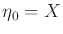, which can be represented as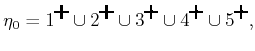(12.14)

the collection of all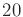states in. Suppose that the action sequence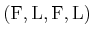is applied. In each case, a motion occurs, which results in the observation history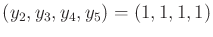.

After the first action,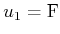, the history I-state is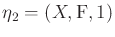. The nondeterministic I-state is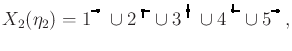(12.15)

which means that any position is still possible, but the successful forward motion removed some orientations from consideration. For example,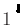is not possible because the previous state would have to be directly south of, which is an obstacle.

After the second action,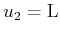,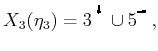(12.16)

which yields only two possible current states. This can be easily seen in Figure 12.2a by observing that there are only two states from which a forward motion can be followed by a left motion. The initial state must have been either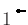or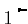.

After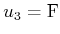is applied, the only possibility remaining is that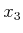must have been. This yields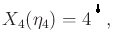(12.17)

which exactly localizes the robot: It is at position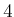facing north. After the final action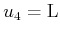is applied it is clear that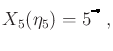(12.18)

which means that in the final state,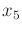, the robot is at positionfacing west. Once the exact robot state is known, no new uncertainty will accumulate because the effects of all actions are predictable. Although it was not shown, it is also possible to prune the possible states by the execution of actions that do not produce motions.Example 12..2 (A Problem that Involves Symmetries)   Now extend the map from Figure 12.2a so that it forms a loop as shown in Figure 12.2b. In this case, it is impossible to determine the precise location of the robot. For simplicity, consider only actions that produce motion (convince yourself that allowing the other actions cannot fix the problem).

Suppose that the robot is initially in positionfacing east. If the action sequence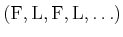is executed, the robot will travel around in cycles. The problem is that it is also possible to apply the same action sequence from position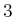facing north. Every action successfully moves the robot, which means that, to the robot, the information appears identical. The other two cases in which this sequence can be applied to travel in cycles are 1) from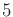heading west, and 2) from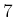heading south. A similar situation occurs from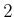facing east, if the sequenceis applied. Can you find the other three starting states from which this sequence moves the robot at every stage? Similar symmetries exist when traveling in clockwise circles and making right turns instead of left turns.

The state space for this problem contains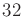states, obtained from four directions at each position. After executing some motions, the nondeterministic I-state can be reduced down to a symmetry class of no more than four possible states. How can this be proved? One way is to use the algorithm that is described next.Steven M LaValle 2020-08-14